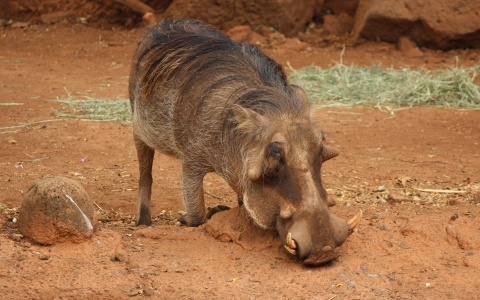This wallpaper features a warthog, the type that the lovable Pumbaa, from Disney's The Lion King, was modeled after. This guy's picture was taken at the the Honolulu Zoo in Hawaii. Warthog males can be up to 4.9ft (1.5m) in length and stand at the shoulder 33in (85cm). They can weigh up to 330lbs (150kg).

Image copyright ©: FreeComputerWallpapers.net Do not use on another site without permission.### function roundNumber(b,c){var a=Math.round(b*Math.pow(10,c))/Math.pow(10,c);return a}function resPrints(){var b=screen.width;var c=screen.height;var a=roundNumber(b/c,2);var d="Your display Resolution: <strong> "+b+" x "+c+" </strong> | Aspect Ratio:<strong> ";if(a==1.25||a==1.33){d+="Standard 4:3"}else{if(a==1.6||a==1.67){d+="Wide 8:5"}else{if(a==1.78){d+="HD 16:9"}else{if(a==1.76){d+="Mobile PSP"}else{if(a==0.75||(a==1.33&&b<=640)){d+="Mobile VGA"}else{if(a==0.6||(a==1.67&&b<=800)){d+="Mobile WVGA"}else{if(a==0.67||(a==1.5&&b==480)){d+="Mobile iPhone"}else{if(a==0.8){d+="Mobile Phone"}else{d="Unknown"}}}}}}}}d+="</strong>";document.write(d)}; resPrints();

Wallpaper details:
Added: Tuesday, 14 August 2012 14:48